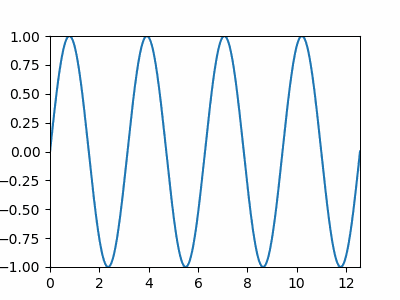# Plot Data in Real Time Using Matplotlib

To plot data in real-time using Matplotlib, or make an animation in Matplotlib, we constantly update the variables to be plotted by iterating in a loop and then plotting the updated values. To view the updated plot in real-time through animation, we use various methods such as `FuncAnimation()` function, `canvas.draw()` along with `canvas_flush_events()`.

## `FuncAnimation()` Function

We can update the plot in real-time by updating the variables `x` and `y` and then displaying updates through animation using `matplotlib.animation.FuncAnimation`.

Syntax:

``````matplotlib.animation.FuncAnimation(fig,
func,
frames=None,
init_func=None,
fargs=None,
save_count=None,
*,
cache_frame_data=True,
**kwargs)
``````

Code:

``````import numpy as np
import matplotlib.pyplot as plt
from matplotlib.animation import FuncAnimation

x = []
y = []

figure, ax = plt.subplots(figsize=(4,3))
line, = ax.plot(x, y)
plt.axis([0, 4*np.pi, -1, 1])

def func_animate(i):
x = np.linspace(0, 4*np.pi, 1000)
y = np.sin(2 * (x - 0.1 * i))

line.set_data(x, y)

return line,

ani = FuncAnimation(figure,
func_animate,
frames=10,
interval=50)

ani.save(r'animation.gif', fps=10)

plt.show()

````````````ani = FuncAnimation(figure,
func_animate,
frames=10,
interval=50)
``````

`figure` is the figure object whose plot will be updated.

`func_animate` is the function to be called at each frame. Its first argument comes from the next value `frames`.

`frames=10` is equal to `range(10)`. Values from 0 to 9 is passed to the `func_animate` at each frame. We could also assign an interalbe to `frames`, like a list `[0, 1, 3, 7, 12]`.

`interval` is the delay between frames in the unit of `ms`.

``````ani.save('animation.gif', fps=10)
``````

We could save the animation to a `gif` or `mp4` with the parameters like `fps` and `dpi`.

## `canvas.draw()` Along With `canvas_flush_events()`

We can update the plot in real-time by updating the variables `x` and `y` with `set_xdata()` and `set_ydata()` and then displaying updates through animation using `canvas.draw()`, which is a method based on JavaScript.

``````import numpy as np
import time
import matplotlib.pyplot as plt

x = np.linspace(0, 10, 100)
y = np.cos(x)

plt.ion()

figure, ax = plt.subplots(figsize=(8,6))
line1, = ax.plot(x, y)

plt.title("Dynamic Plot of sinx",fontsize=25)

plt.xlabel("X",fontsize=18)
plt.ylabel("sinX",fontsize=18)

for p in range(100):
updated_y = np.cos(x-0.05*p)

line1.set_xdata(x)
line1.set_ydata(updated_y)

figure.canvas.draw()

figure.canvas.flush_events()
time.sleep(0.1)

``````Here the values of `x` and `y` get updated repeatedly and the plot also gets updated in real time.

`plt.ion()` turns on the interactive mode. The plot will not be updated if it is not called.

`canvas.flush_events()` is method based on JavaScript to clear figures on every iterations so that successive figures might not overlap.

## Real Time Scatter Plot

However, to make a real-time scatter, we can just update the values of `x` and `y` and add scatter points in each iteration. In this case, we need not clear every figure as a scatter plot generally represents a distinct point in the plane and the points have very little chance of overlapping.

``````import numpy as np
import matplotlib.pyplot as plt
x=0
for i in range(100):
x=x+0.04
y = np.sin(x)
plt.scatter(x, y)
plt.title("Real Time plot")
plt.xlabel("x")
plt.ylabel("sinx")
plt.pause(0.05)

plt.show()
``````Write for us
DelftStack articles are written by software geeks like you. If you also would like to contribute to DelftStack by writing paid articles, you can check the write for us page.